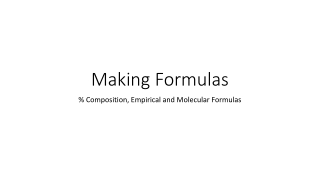# Making Formulas - PowerPoint PPT PresentationDownload PresentationMaking Formulas

Making Formulas
Download Presentation## Making Formulas

- - - - - - - - - - - - - - - - - - - - - - - - - - - E N D - - - - - - - - - - - - - - - - - - - - - - - - - - -
##### Presentation Transcript

1. Making Formulas % Composition, Empirical and Molecular Formulas

2. Percent Composition Percent composition of a compound refers to the percentage by mass.  • In other words, the percentage composition of water shows what % (by mass) is Hydrogen, and what % is Oxygen. Percentage composition by mass does not tell us the ratio by which the atoms combine. (Does not tell us the subscripts of chemical formulas)

3. % Composition Example Jim goes to a school that has 258 total students.  If 164 of the students are boys, what percentage of the student body is made up of girls?

4. % Example In this example, you found the percent composition by dividing one part by the total and multiplying by 100. part % composition = -------------- x 100       whole % Girls = (258-164) x 100 258 % Girls = 36.4

5. Percent Composition Finding the percentage composition by mass works the same way.  • Divide the mass that one element contributes to the compound by the mass of the entire compound.  mass from element % Composition = --------------------------- x 100total mass of the compound

6. % Composition Example What percentage of the mass of carbon dioxide (CO2) is made up by the carbon? Mass of Carbon = 12.01 x 1 = 12.01 g Mass of Oxygen = 16.00 x 2 = 32.00 g part % comp. of Carbon = --------------- x 100 whole 12.01 g % comp. of Carbon = --------------- x 100 44.01 g Answer = 27.3%

7. % Composition Example What is the percentage composition of carbon in glucose (C6H12O6) ?

8. Percent Composition and Formulas • Percent composition only tells you what percentage of the compound that a particular atom is. It doesn’t tell you how many atoms are in that compound. • HOWEVER, it does help you to determine that compound’s empirical formula.

9. Empirical Formula The simplest whole number ratio of atoms in a compound

10. Empirical Formulas Identify each of the following as empirical or molecular: • CH4 • H2O • C2H6 • NaOH • C6H12O6

11. Empirical Formulas Identify each of the following as empirical or molecular: • CH4 - Empirical • H2O - Empirical • C2H6 - Molecular • NaOH- Empirical • C6H12O6 - Molecular

12. Steps to Calculate Empirical Formulas • Convert all given masses to moles (so they can be compared) • Find the lowest whole number ratio in moles by dividing by the smallest number of moles. • This whole # value becomes the subscript. The subscripts in the formula will reflect the smallest whole number ratio between the moles of each of the elements present.

13. Example A sample of urea is decomposed into its elements. The following products are measured: Nitrogen: 1.121 g Hydrogen: 0.161 g Carbon: 0.480 g Oxygen: 0.640 g

14. 1. Find the number of moles of each element present: Nitrogen: 1.121 g x ( )= hydrogen: .161 g x ( )= carbon: .480 g x ( )= oxygen: .640 g x ( )= 0.08 mol N 0.16 mol H 0.04 mol C 0.04 mol O

15. 0.08 mol N 0.16 mol H 0.04 mol C 0.04 mol O / 0.04 mol = / 0.04 mol = / 0.04 mol = / 0.04 mol = 2.0 N 4.0 H 1.0 C 1.0 O 2. Find the lowest whole number ratio in moles (the empirical formula)

16. 2.0 N 4.0 H 1.0 C 1.0 O N2H4CO 3. The subscripts in the formula will reflect the smallest whole number ratio between the moles of each of the elements present.

17. Practice Problem What is the empirical formula for a compound which contains 0.0134 g of iron, 0.00769 g of sulfur and 0.0115 g of oxygen?

18. Steps to Calculate Molecular Formulas

19. Practice Problem A compound is 92.24% Carbon and 7.76% Hydrogen. The molar mass of the molecular compound is 78.1 g. Calculate the empirical formula and molecular formula of the compound.

20. The strange case of Mole Airlines Flight 1023

21. Mole Airlines Flight 1023 • Scenario: Read with your partner • Your Job: • Determine empirical formulas (Table 3) • Match your empirical formulas with the substances listed in Table 1 • Compare the completed Table 3 with Table 2 to complete the Victim Identification Form • Identify the murderer, victim, and explosive substance.